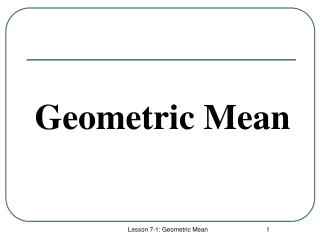Download PresentationGeometric Mean

# Geometric Mean - PowerPoint PPT PresentationDownload Presentation## Geometric Mean

- - - - - - - - - - - - - - - - - - - - - - - - - - - E N D - - - - - - - - - - - - - - - - - - - - - - - - - - -
##### Presentation Transcript

1. Geometric Mean Lesson 7-1: Geometric Mean

2. Sequences Arithmetic Sequence: Is a pattern of numbers where any term (number in the sequence) is determined by adding or subtracting the previous term by a constant called the common difference. 17 20 23 Example: 2, 5, 8, 11, 14, ____, ____, ____ Common difference = 3 Geometric Sequence: Is a pattern of numbers where any term (number in the sequence) is determined by multiplying the previous term by a common factor. Example: 2, 6, 18, 54, 162, _____, _____, ____ 486 1458 4374 Common Factor = 3 Lesson 7-1: Geometric Mean

3. Examples 1. Starting with the number 1 and using a factor of 4, create 5 terms of a geometric sequence. 1 , 4 , 16 , 64 , 256 2. Starting with the number 2 and using a factor of 5, create 5 terms of a geometric sequence. 2 , 10 , 50 , 250 , 1250 3. Starting with the number 5 and using a factor of 3, create 5 terms of a geometric sequence. 5 , 15 , 45 , 135 , 405 4. In the geometric sequence 2, ____, 72, 432, .Find the missing term. 12 12 5. In the geometric sequence 6, ____, 24,... Find the missing term. Lesson 7-1: Geometric Mean

4. Geometric Mean A term between two terms of a geometric sequence is the geometric mean of the two terms. Example: In the geometric sequence 4, 20, 100, ….(with a factor of 5), 20 is the geometric mean of 4 and 100. Try It: Find the geometric mean of 3 and 300. 3 , ___ , 300 30 Lesson 7-1: Geometric Mean

5. Geometric Mean : Fact Consecutive terms of a geometric sequence are proportional. Example: Consider the geometric sequence with a common factor 10. 4 , 40 , 400 (4)(400) = (40)(40) cross-products are equal 1600 = 1600 Lesson 7-1: Geometric Mean

6. Therefore ……….. To find the geometric mean between 7 and 28 ... label the missing term x 7 , ___ , 28 write a proportion cross multiply solve Lesson 7-1: Geometric Mean

7. The geometric mean between two numbers a and b is the positive number x where . Therefore x = . Try It: Find the geometric mean of . . . Answer = 20 1. 10 and 40 2. 1 and 36 Answer = 6 3. 10 and 20 Answer = 14.14 4. 5 and 6 Answer = 5.48 Answer = 9.94 5. 8.1 and 12.2 Lesson 7-1: Geometric Mean

8. How does this relate to geometry? Lesson 7-1: Geometric Mean

9. The " W " Pattern Lesson 7-1: Geometric Mean

10. The Geometric Means Recall the three geometric means that you discovered from your Sketchpad activity. BUT FIRST . . . Lesson 7-1: Geometric Mean

11. Re-label the Sides (as lengths) Lesson 7-1: Geometric Mean

12. Geometric Mean #1 What is the proportion that uses f? f is the geometric mean of d and e. Lesson 7-1: Geometric Mean

13. Geometric Mean #2 What is the proportion that uses b? b is the geometric mean of e and c. Lesson 7-1: Geometric Mean

14. Geometric Mean #3 What is the proportion that uses a? a is the geometric mean of d and c. Lesson 7-1: Geometric Mean

15. Put them all together Lesson 7-1: Geometric Mean

16. The “W” Pattern W Lesson 7-1: Geometric Mean

17. Try it ! Given: d = 4 and e = 10 Find: a = ___ b = ___ c = ___ f = ___ Lesson 7-1: Geometric Mean

18. Solution: Proportions Answers Lesson 7-1: Geometric Mean